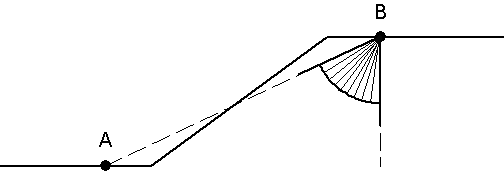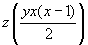Join one of our upcoming courses taking place around the world! Explore now

# Auto Refine Search

The Auto Refine Search is one of the Search Methods available in Slide2 for locating the Global Minimum safety factor for CIRCULAR slip surfaces. In order to perform an Auto Refine Search, the Surface Options dialog must be configured as follows:

• Surface Type = Circular
• Search Method = Auto Refine Search
The Auto Refine Search method can also be applied to non-circular surfaces, see the Auto Refine (Non-Circular) topic.

## How Does the Auto Refine Search Work?

With the Auto Refine Search method, the search for the lowest safety factor is refined as the search progresses. An iterative approach is used, so that the results of one iteration, are used to narrow the search area on the slope in the next iteration.

The Auto Refine Search method works as follows:

1. First, the slope surface, as defined by the Slope Limits, is divided into a number of divisions, according to the value of the Divisions Along Slope.
2. Circles are then generated between EACH PAIR OF DIVISIONS, according to the value of the Circles Per Division (see below for illustration).
3. The safety factors are then calculated for these circles, and the average safety factor associated with EACH DIVISION along the slope, is recorded.
4. This constitutes ONE ITERATION of the Auto Refine Search method.
5. The value of Divisions To Use In Next Iteration is then used to determine how many divisions along the slope, will be used to define a new, narrowed search area, in the next iteration. Only the divisions with the lowest (average) safety factors are used, the divisions with high safety factors are discarded from the analysis.

FOR EXAMPLE:

• If the Divisions Along Slope = 10
• Divisions To Use In Next Iteration = 50%
• Then after each iteration, 5 divisions (with the lowest average safety factors) will be used, and 5 (with higher safety factors) will be discarded.
6. The divisions of the slope which are retained, are then used to form a new slope polyline. Using this new, narrowed slope surface, steps 1 to 5 are then repeated, for the Number of Iterations specified.

The Auto Refine Search method is a simple but effective algorithm, for locating the Global Minimum circular slip surface. In many cases, for an equal number of surfaces generated, the Auto Refine method will locate lower safety factor surfaces than a Grid Search or a Slope Search.

The parameters of the Auto Refine Search method, entered in the Surface Options dialog, are used as follows.

## Divisions Along Slope

The Divisions Along Slope is the number of divisions into which the slope surface is divided, FOR EACH ITERATION OF THE SEARCH.

• For the initial iteration of the search, the slope surface is defined by the Slope Limits.
• For each subsequent iteration, the slope surface to be analyzed, is defined by the value of Divisions to Use in Next Iteration (see below).
The Divisions Along Slope are always measured ALONG THE SLOPE POLYLINE. They are NOT measured horizontally. This means that the analysis is not dependent on the angle of the slope segments.

## Circles Per Division

This determines how many slip circles are generated, between EACH PAIR OF DIVISIONS along the slope. The circles are generated as follows.

1. For each pair of divisions along the slope, a straight line joining the midpoints of the divisions, is determined.
2. The slope of this line determines a MINIMUM angle.
3. A vertical line (90 degrees) determines a MAXIMUM angle.
4. The angular range between the MINIMUM angle and the MAXIMUM angle, is then divided equally according to the number of Circles Per Division.
5. A small offset is actually used from the MINIMUM and MAXIMUM angles, so that valid circles are generated.

How circles are generated between each pair of divisions in Auto Refine Search.6. Using these initial tangent angles, circles are then generated between each pair of divisions, as shown above.

## Number of Iterations

The Number of Iterations used for the Auto Refine Search.

The analysis is always carried out for the specified Number of Iterations. That is, there is no "convergence" or "cutoff" criteria, the total number of surfaces and iterations will always be generated.

## Divisions to Use in Next Iteration

The number of divisions along the slope, with the lowest safety factors, is to be used as the basis for the next iteration in the Auto Refine Search. (See the description above for an example).

## Number of Circles Generated

In the dialog, as you enter the Auto Refine Search parameters, the number of surfaces which will be created, is displayed in the dialog. This number is determined as follows.

If:

Divisions Along Slope = x

Circles Per Division = y

Number of Iterations = z

then:

Number of circles generated PER ITERATION =Total number of circles generated =The default values of x, y and z (10, 10, 10) often give good results. (This generates 4500 circles). As you can see from the above formulae, the number of circles generated, is proportional to the SQUARE of the number of Divisions Along Slope, so you should not enter a large value of Divisions Along Slope, or the total number of circles generated will become very large. Remember that the iteration process takes care of the search refinement, and that the search area is always decreasing. Therefore it is not necessary to use a large number of Divisions Along Slope.

The number of Divisions to Use in the Next Iteration, DOES NOT affect the number of surfaces generated. The default value of 50% is recommended. However, if you experiment with changing this value, you will find that the final results are not very sensitive to this parameter.

## Other Search Options

For information about the following Auto Refine Search options:

• Composite Surfaces

Please see the Grid Search topic, as the details are the same.

The Create Tension Crack for Reverse Curvature option is not available for the Auto Refine Search Method, because of the way in which the circles are generated (i.e. within the MINIMUM and MAXIMUM angles described above, it is not possible to generate a reverse curvature surface).

## Surface Filter

See the Surface Options topic for details about the Minimum Elevation, Minimum Depth, Minimum Area, Minimum Weight slip surface filter options.